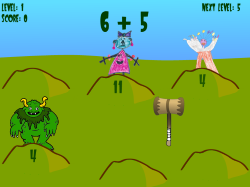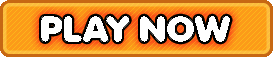Bookmark

# WhackATroll Basic Math Facts Addition, Subtraction, Multiplication, Division

4 stars (613 plays / 3 votes)Whack-A-Troll | Basic Math Facts (Addition, Subtraction, Multiplication, & Division)Basic facts game for kids that requires hitting the troll with the correct answer with a mallet.
4 stars out of 5 (613 plays / 3 votes)

KidzSearch > Games > Math GamesTags:  math    basic    fact    addition    addition    subtraction    multiplication    multiply    division    divide    problem    elementary    math    number    senseMember of the American Library Association  Copyright 2005-2020 KidzSearch.com